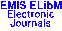Publications de l'Institut Mathématique, Nouvelle Série Vol. 82(96), pp. 141–154 (2007)

Previous Article

Next Article

Contents of this Issue

Other Issues

ELibM Journals

ELibM Home

EMIS Home

Pick a mirror

## A LOGIC WITH HIGHER ORDER CONDITIONAL PROBABILITIES

### Zoran Ognjanovic and Nebojsa Ikodinovic

Matematicki institut, Kneza Mihaila 36, 11000 Beograd p.p. 367, Serbia and Prirodno-matematicki fakultet, 34000 Kragujevac, Serbia

Abstract: We investigate probability logic with the conditional probability operators. This logic, denoted $LCP$, allows making statements such as: $P_{\geq s}\alpha$, $CP_{\geq s}(\alpha\mid\beta)$, $CP_{\leq 0}(\alpha\mid\beta)$ with the intended meaning "the probability of $\alpha$ is at least $s$", "the conditional probability of $\alpha$ given $\beta$ is at least $s$", "the conditional probability of $\alpha$ given $\beta$ at most $0$". A possible-world approach is proposed to give semantics to such formulas. Every world of a given set of worlds is equipped with a probability space and conditional probability is derived in the usual way: $P(\alpha\mid\beta)=\frac{P(\alpha\wedge\beta)}{P(\beta)}$, $P(\beta)>0$, by the (unconditional) probability measure that is defined on an algebra of subsets of possible worlds. Infinitary axiomatic system for our logic which is sound and complete with respect to the mentioned class of models is given. Decidability of the presented logic is proved.

Classification (MSC2000): 68T37; 68T27; 03B48; 03B70

Full text of the article: (for faster download, first choose a mirror)